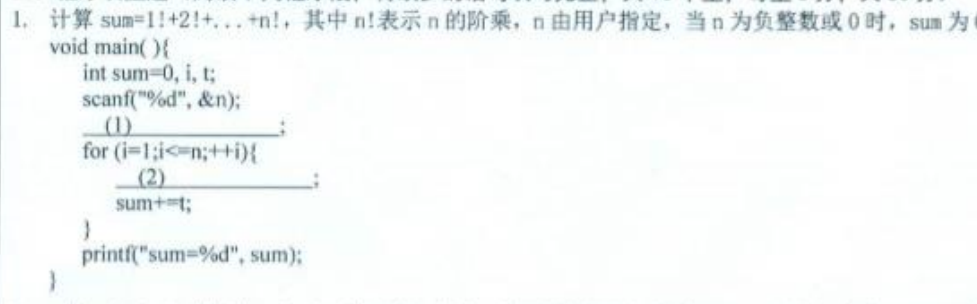2021-11-22 15:37

第二个空怎么填？难道有直接求阶乘的库函数int sum=0,i, t;
scanf("%d", &n);
for (i=1;i<=n;++i){

sum+t;
}
print("sum=%d", sum);
)

• 写回答
• 好问题 提建议
• 追加酬金
• 关注问题
• 收藏
• 邀请回答